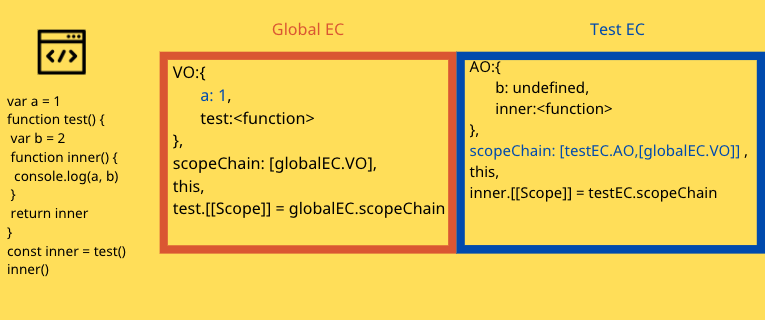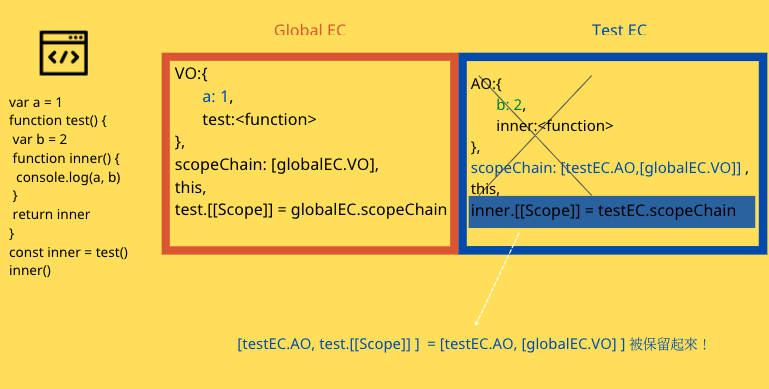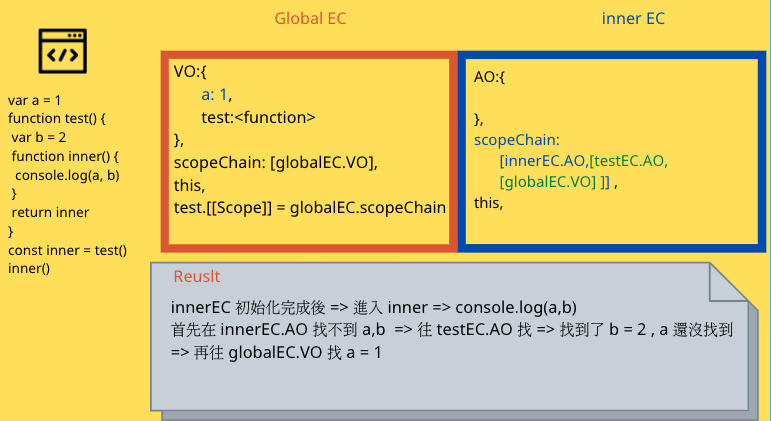# JavaScript 程式執行原理：Closure

## Closure

var a = 1
function test() {
var b = 2
function inner() {
console.log(a, b) // 1 2
}
return inner
}
const inner = test()
inner()global EC => 執行 => testEC => 執行 ：[testEC.AO, test.[[Scope]] ] = [testEC.AO, [globalEC.VO] ] 被保留起來！function complex(n) {
console.log('進入運算')
return n*n*n
}

function cache(fn){
var ans = {}
return function(n) {
if(ans[n]) {
return ans[n]
}
ans[n] = fn(n)
return ans[n]
}
}

const cachedComplex = cache(complex)

console.log(cachedComplex(10)) // 進入運算 1000
console.log(cachedComplex(10)) // 1000
console.log(cachedComplex(10)) // 1000
console.log(cachedComplex(10)) // 1000


var arr = []
for (var i =0; i< 5; i++){
arr[i] = function(){
console.log(i)
}
}

arr()


var arr = []
for (var i =0; i< 5; i++){
arr[i] = logN(i)
}

function logN(n) {
return function(){
console.log(n)
}
}
arr()


var arr = []
for (var i =0; i< 5; i++){
arr[i] = (function(n){
return function(){
console.log(n)
}
})(i)
}

arr()


### closure 的應用

var money = 99
money += num
}
function deduct(num) {
if(num >=10) {
money -=10
} else {
money -=num
}
}
deduct(100)
console.log(money) //90


function createWallet(initMoney){
var money = initMoney
return {
money +=num
},
deduct: function(num){
if(num >=10) {
money -=10
} else {
money -=num
}
},
getMoney() {
return money
}
}
}

var  myWallet = createWallet(99)
myWallet .deduct(100)
console.log(myWallet.getMoney()) //90